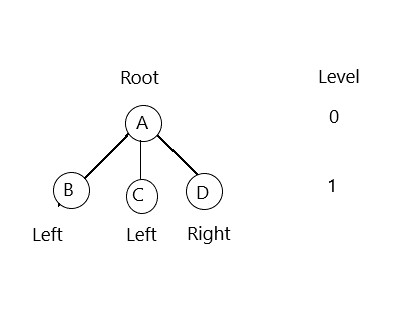## Latest:

latest

### Here is discussed Pre-order, In order, and post-order Dfs algorithm  ( Depth First Search ) in AI with a single example.  Learn the BFS algo...

Here is discussed Pre-order, In order, and post-order Dfs algorithm ( Depth First Search ) in AI with a single example.

Learn the BFS algorithm

## Dfs algorithm

In the problem, you will see a tree is given. There will be a goal node also. You have to go to the goal node from the root node by using the Depth-first search. The node in level 0(Before that level there is no edge) is called the root node.Rules to follow:

• See the given goal node
• Leveling
• Start Visiting from the root node and then
• If you find the goal node then stop searching
1. Preorder: Root>Left>Right
2. Inorder: Left>Root > Right
3. Postorder: Left> Right>Root
For this example

### Preorder Dfs algorithm

Root>Left>Right

Rule: At first we have to complete all the root nodes then the left and at last the right nodes.

Here,

At first, we see, F is the root. For root F, D is the left node and J is the right. So, we have to next to the left node D.

F>D

Now D is a root and here B is left. So,

F>D>B

For root B left is A and right is C. So,

F>D>B>A>C

We had a right node for root D. So,

F>D>B>A>C>E

So far all the nodes from the left subtree are visited. Now we have to visit the right subtree.

At first in the right, we found root J. So,

F>D>B>A>C>E>J

Now J is a root and its left is G. So,

F>D>B>A>C>E>J>G

Now G is a root. Here is no left node but has a right I. So,

F>D>B>A>C>E>J>G>I

Now, I is a root and it's left node is H. So,

F>D>B>A>C>E>J>G>I>H

We have visited all the left node of root J. Now we have to visit the right of the root J. There is a node K which has no left or right node. So, finally, we got after visiting,
• Preorder DFS: F>D>B>A>C>E>J>G>I>H>K

### In order Dfs algorithm

Left>Root > Right

Rule: At first we have to complete all the left node then the root and at last the right nodes.

Here F is a root and it's left is D. Now D is a root and it's left is B, B is a root also and A is the left here.

Now A is the leftmost node. So we have to start from here.

A is left, its root is B and right C. So,

A>B>C

Now, for left node B root is D and right is E. So,

A>B>C>D>E

Now F is the root of the left subtree. So,

A>B>C>D>E>F

Now, J is the right node of F. But next, it has left node G. So,

A>B>C>D>E>F>G

G has no left node but a right node I.

A>B>C>D>E>F>G>H>I

Now left subtree of root node J is completed. so,

A>B>C>D>E>F>G>H>I>J

J has a right node K and it has no left or right, and it is the rightmost node. So finally we got,
• In order DFS: A>B>C>D>E>F>G>H>I>J>K

### Postorder Dfs algorithm

Left> Right>Root

At first, we have to to the most left node. From the graph we can see, A is the most left node. So we have to start from here. B is the root and C is right. So,

A > C> B

Now B is left for root D and E is the Right. So,

A>C>B>E>D

Now left subtree of root F is completed. Next, we have to visit the right. In the right H is the most left node. So,

A>C>B>E>D>H

I is the root of H. So,

A>C>B>E>D>H>I

G is the root of I, So,

A>C>B>E>D>H>I>G

Nw J is the root of left node G and right node K. At first, we have to visit K(right). So,

A>C>B>E>D>H>I>G>K

Now we have to visit the root of G and K which is J. So,

A>C>B>E>D>H>I>G>K>J

We have visited the left and right subtree for the root F. So, now we have to visit the root ( F ) finally. At last, we have got.
• Postorder DFS: A>C>B>E>D>H>I>G>K>J>F
So, these are Pre-order, In order, and post-order Dfs algorithm ( Depth First Search ) with a single example.